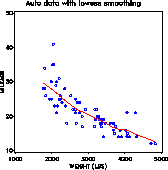lowess lowess - LOcally Weighted robust Scatterplot Smoothing lowess

# SAS Macro Programs: lowess

\$Version: 2.2 (26 Jan 2005)
Michael Friendly
York University

## LOWESS macro (get lowess.sas)

The LOWESS macro performs robust, locally weighted scatterplot smoothing as described in "Section 4.4.2". The data and the smoothed curve are plotted if PLOT=YES is specified. The smoothed response variable, residuals, and observation weights are returned in the output data set named by the OUT= parameter. An optional output ANNOTATE= data set can also be produced, which may be used to apply the lowess smoothing in a more complex plotting application.

As of Version 2.1, the LOWESS macro will use PROC LOESS if running under Version 7 of the SAS System. This makes the macro much faster, particularly for large data sets. For use with SAS 6.12 or earlier, use the STEP= parameter to control the number of data points at which the local regressions are computed.

### Parameters

DATA=_LAST_
Name of the input data set.
X = X
Name of the independent (X) variable.
Y = Y
Name of the dependent (Y) variable to be smoothed.
ID=
Name of an optional character variable to identify observations.
OUT=SMOOTH
Name of the output data set. The output data set contains the X=, Y=, and ID= variables plus the variables _YHAT_, _RESID_, and _WEIGHT_. _YHAT_ is the smoothed value of the Y= variable, _RESID_ is the residual, and _WEIGHT_ is the combined weight for that observation in the final iteration.
F = .50
Lowess window width, the fraction of the observtions used in each locally-weighted regression. A larger window width makes the curve smoother, but may lead to lack of fit. Values of F > 1 are allowed.
P = 1
Degree of the locally-weighted regressions. P=1 gives linear fits, appropriate when the data do not have several peaks and valleys; P=2 gives quadratic fits, which are useful when they do.
ITER=2
Total number of iterations.
ROBUST=1
Specifies whether to perform robustness re-weightings, which decrease the weights for observations with large residuals in the next iteration. Set ROBUST=0 to suppress the robust calculations. For binary dependent variables, the robustness step is usually not performed.
CLM=
[Version 7+ only] Specifies the significance level for confidence intervals about the smoothed curve. Use CLM=0.05 for 95% confidence intervals.
STEP=1
Step for successive X values. By default, the macro performs the locally-weighted regression at each X[i], which can be computationally intensive for moderately large data sets. Setting STEP > 1 causes the macro to perform the regression at every STEP-th value of the index i, and to use predicted values from that regression for intermediate points. It is recommended to specify a STEP value at least n/100 for moderate to large sized datasets.
PLOT=NO
Specifying PLOT=YES, draws both a printer plot and a high-resolution plot. You may wish to change the default values of the PLOT, GPLOT or PPLOT options to suit your taste.
GPLOT=NO
Draw the plot? If you specify PLOT=YES, a high-resolution plot is drawn by the macro.
PPLOT=NO
Draw a printer plot? If you specify PPLOT=YES, a printer plot is drawn by the macro.
SYMBOL=CIRCLE
Plotting symbol used for points
HTEXT=1.5
Height for axis labels and values
HSYM=1.5
Height for point symbols
COLORS=BLACK RED
colors for points and smooth curve
LINE=1
Line style for the smooth curve
HAXIS=
The name of an AXIS statements for the horizontal axis
VAXIS=
The name of an AXIS statements for the vertical axis
OUTANNO=
Name of output ANNOTATE= dataset which draws the smoothed lowess curve. This dataset is produced only if the OUTANNO= name is specified.
IN=
Name of an optional input ANNOTATE= data set, which is concatenated to the OUTANNO= data set.
NAME=LOWESS
The name assigned to the graph in the graphic catalog.

### Missing data

Any observations with missing data on the X or Y variables are removed before finding the lowess fit.

### Usage Note

Under some older versions of the SAS System, it may be neccessary to add the option WORKSIZE=100 to the PROC IML statement.

### Example

This example plots gas mileage (MPG) vs weight, with a smoothed lowess curve showing a (slight) nonlinear dependence of mileage on weight. The plot is drawn by the macro from the OUT=SMOOTH data set. An output ANNOTATE= data set is also produced.
```title 'Auto data with lowess smoothing';
%include data(auto);
%include macros(lowess);

%lowess(data=auto,out=smooth, x=weight, y=mpg,
id=model, htext=2,
f=.4, plot=YES,
colors=blue red, outanno=lowess);

```